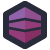Nedra writes “I discovered this code while cleaning up and refactoring some label printing methods in a home-grown ERP that I maintain.”

The code in question “works most of the time”, which means it’s crossed a line of code quality. Speaking of crossing lines, this particular block of code needs to take information about how a product is formulated and print it on a label. These sorts of ERP functions are “mission critical”, in that correct and accurate formulations- whether the ingredients list on a foodstuff or the ingredients in a can of paint, or an industrial solvent- are required for regulatory compliance.

Labels are also physical objects, and have a defined physical size. This means that you can only fit so much information on them, and you’ll need to make sure the layout of what you’re printing is readable on the label.

Nedra’s co-worker had… a solution for this.

``````  Dim result As String = ""
Dim f As String() = Formula.Split(New String() {Environment.NewLine}, StringSplitOptions.None)
If f.Count > 8 Then
For Each line In f
If line.Length > 80 Then
Dim break As Integer = line.Substring(0, 80).LastIndexOf(" ") + 1
line = line.Insert(break, Environment.NewLine)
If line.Length > 161 Then
break = line.Substring(0, 161).LastIndexOf(" ") + 1
line = line.Insert(break, Environment.NewLine)
If line.Length > 242 Then
break = line.Substring(0, 242).LastIndexOf(" ") + 1
line = line.Insert(break, Environment.NewLine)
If line.Length > 323 Then
break = line.Substring(0, 343).LastIndexOf(" ") + 1
line = line.Insert(break, Environment.NewLine)
End If
End If
End If
End If
If String.IsNullOrEmpty(result) Then
result = line
Else
result += Environment.NewLine + line
End If
Next
Else
For Each line In f
If line.Length > 65 Then
Dim break As Integer = line.Substring(0, 65).LastIndexOf(" ") + 1
line = line.Insert(break, Environment.NewLine)
If line.Length > 131 Then
break = line.Substring(0, 131).LastIndexOf(" ") + 1
line = line.Insert(break, Environment.NewLine)
If line.Length > 197 Then
break = line.Substring(0, 197).LastIndexOf(" ") + 1
line = line.Insert(break, Environment.NewLine)
If line.Length > 263 Then
break = line.Substring(0, 263).LastIndexOf(" ") + 1
line = line.Insert(break, Environment.NewLine)
End If
End If
End If
End If
If String.IsNullOrEmpty(result) Then
result = line
Else
result += Environment.NewLine + line
End If
Next
End If``````

After writing a block like this, you definitely need to take a break. At its core, this code injects linebreaks at specific positions in a string depending on how many total original lines there were. For a long moment, I was trying to figure out the off-by-one errors that it looked like it had- 80 characters, then 161?- but that’s specifically because it’s inserting characters.

It’s not efficient, it’s not easy to read, it’s not easy to skim, but it does work. Mostly. Some formulas have a lot of content, which means this break pattern doesn’t always actually fit the content correctly.[Advertisement] Otter - Provision your servers automatically without ever needing to log-in to a command prompt. Get started today!